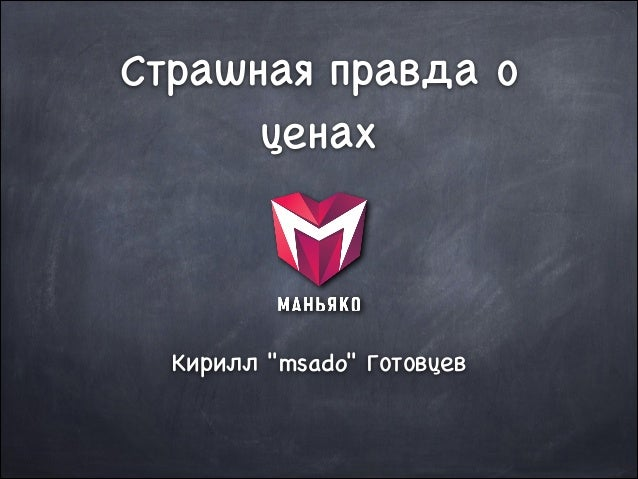Successfully reported this slideshow.
We use your LinkedIn profile and activity data to personalize ads and to show you more relevant ads. You can change your ad preferences anytime.Upcoming SlideShare
Loading in …5
×

# Сколько стоит web-сайт

Не забываем после слайдов сходить на http://bit.ly/2cTE6LR - там лежат другие лекци

• Full Name
Comment goes here.

Are you sure you want to Yes No
Your message goes here• Login to see the comments

### Сколько стоит web-сайт

1. 1. Страшная правда о ценах Кирилл "msado" Готовцев
2. 2. А давайте посчитаем деньги всерьез?
3. 3. С начала посчитаем прямые расходы
4. 4. Прикинем ставки, причем не московские Люди попроще 160 x 200 = 32 000 Специалисты 160 x 300 = 48 000 Ключевые специалисты 160 x 400 = 64 000
5. 5. детские деньги, да?
6. 6. Проектирование Аналитика 40 x 200 = 8 000 Прототипы 50 x 200 = 10 000 Юзабилити 20 x 300 = 6 000 Базовый дизайн 80 x 400 = 32 000 Итого 8 + 10 + 6 + 32 = 56 тысяч
7. 7. Все ли это расходы на проектирование?
8. 8. Согласования по проектированию Аналитика 20 x 300 = 6 000 Прототипы 10 x 300 = 3 000 Юзабилити 3,3 x 300 = 1000 Базовый дизайн 20 x 300 = 6 000 Итого 6 + 3 + 1 + 6 = 16 тысяч
9. 9. Менеджмент сейчас не считаем. ! Потом объясню почему
10. 10. Производство Полный дизайн + иллюстрации 100 x 300 = 30 000 Верстка 40 x 300 = 12 000 Движок, прикрутка, 50 x 400 = 20 000 Сборка 10 x 300 = 3 000 Итого 30 + 12 + 20 + 3 = 65 тысяч
11. 11. Кто знает какой этап дальше?
12. 12. Простой в ожидании контента от заказчика ! Фиг с ним, не оцениваем отдельно
13. 13. Контент Подготовка контента 20 x 300 = 6 000 Введение контента в сайт 60 x 200 = 12 000 Вычитка, ОТК 20 x 300 = 6 000 Исправление ошибок 10 x 200 = 2 000 Итого 6 + 12 + 6 + 2 = 26 тысяч
14. 14. Кто знает какой этап дальше?
15. 15. Тестирование Соответствие функциональным требованиям 10 x 300 = 3 000 Согласование с заказчиком 10 x 300 = 3 000 Внесение допустимых корректив 40 x 300 = 12 000 Итого 3 + 3 + 12= 18 тысяч
16. 16. Прямые выплаты Проектирование с согласованием 56 000 + 16 000 = 72 000 Производство 65 000 Контент 26 000 Тестирование 18 000 Итого 72 + 65 + 26 + 18 = 181 тысяча
17. 17. У кого возникло чувство, что я не все посчитал?
18. 18. Я же не посчитал управление всеми этими красавцами!
19. 19. 181 000 + 30% = 235 000
20. 20. Кто в зале знает, что такое OVERHEAD поднимите руки
21. 21. упрощенно: OVERHEAD = расходный бюджет / фот' выраженный в добавленных процентах ! Где фот' - фот производства
22. 22. Я исхожу из среднего по отрасли 120%
23. 23. 235 000 + 120% = 517 000
24. 24. Дальше спорное утверждение, вернее оно может варьироваться сильнее чем все остальное ! Норматив выплат физлицам в расходном бюджете - 80%
25. 25. 517 000 * 80% = 413 000
26. 26. 413 000 это то что мы выдадим по проекту на руки
27. 27. Налоги Подоходный 413 000 * 13% = 53 000 Соцфонды 413 000 * 34% = 140 000 Итого 53 + 140 = 193 тысячи
28. 28. 413 000 + 193 000 = 606 000 у нас получилось суммарно по физлицам
29. 29. Кто знает, почему я привязался к физлицам?
30. 30. Я очень надеюсь, что хоть ктото в зале знает бухгалтерию и сказал ! ВМЕНЕННЫЙ НДС
31. 31. 606 000 * 18% = 109 Вот теперь мы посчитали все налоги на прямые выплаты. ! Посмотрим что у нас получилось
32. 32. 517 000 + 193 000 + 109 000 = 819 000
33. 33. У меня есть сложный идеологический вопрос: ! Кто оплачивает ошибки, простой, взаимонепонимание, запои и прочее, прочее, прочее?
34. 34. РИСКИ ! В среднем разумный объем рисков порядка 20%
35. 35. 819 000 + 20% = 982 000
36. 36. 982 долбаные тысячи СЕБЕСТОИМОСТИ ! а лично мне бы хотелось еще что-то ЗАРАБОТАТЬ
37. 37. Вы заплатили за сайт 500 тысяч? ! прикидывайте, на чем исполнитель резал себестоимость ! Потому что чудес не бывает
38. 38. На этой трагической ноте мы и закончим нашу секцию Сфотографигуйте на телефон ссылочки: www.maniaco.ru msado.livejournal.com facebook.com/kirill.gotovtsev